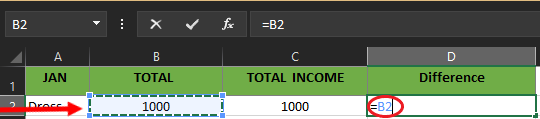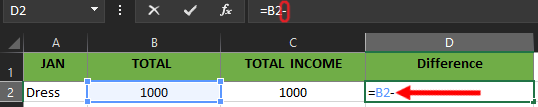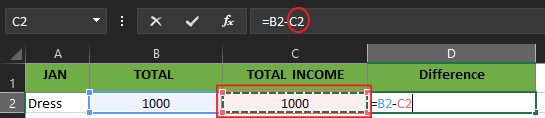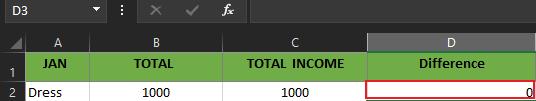# Excel Subtraction Formula (Dates, Time, Cells, Column)

In this topic, we will know what Excel subtraction formula is or if it exists in a specific way. Thus different ways to do it, particularly in your worksheet set of data.

In addition, as we cover this topic you will encounter examples and figures that will help you understand as well as practice it in your manner.

## What is the Subtract Function in Excel

Technically there is no subtraction function in excel however there are a lot of ways to do subtraction in excel. Firstly, let us know what the subtraction operation is…

Subtraction is composed of two parts which is the first number called minuend where a number is subtracted from. Then the second number is called subtrahend which is the number subtracted. The result is called the difference.

Another thing is the ‘minus’ (-) sign is used between the minuend and subtrahend.

Correspondingly, excel also facilitates the subtraction process, which could either numbers, cells, matrices, times, dates and percentages, etc. In addition to this, it uses and begins with an equal sign (=) along with the first number, the minus sign, and the second number.

For instance, you are going to subtract 10 from 20. Therefore it goes like this( =20-10).

## How to Subtract in Excel

In subtraction, you will use the minus sign (-) formula but you will use cell references instead of actual numbers. The first thing you should remember in creating a formula is to put an equal sign to start the formula.
For instance, to subtract numbers of a cell from another cell use this syntax: `=A2-B2`
Here is how to subtract in Excel follow these steps to create yours:

• Step 1. To start your formula type the equal sign(=) where you want to see the result of subtraction.• Step 2. Select the cell you want to be minuend; it is the number where the other number is to be subtracted. Along with it the reference cell is automatically placed in the formula.• Step 3. Type a minus sign (-).• Step 4. Select the cell you want to be subtrahend; it is the number subtracted. Along with it the reference cell is automatically placed in the formula.• Step 5. Press the Enter key to complete your formula.## How to Subtract Columns in Excel

Excel allows subtraction of two columns row by row in the easiest way, to workout this you need to create a formula in the first cell in which you want the operation occurs. Then drag the fill handle to the range of cells or you can also double-click the plus sign and copy the formula.

To visualize here are the figures showing how it has been done.

In the above example, we subtract the first row, where the number in column C is subtracted from column B. Therefore the formula is created as this: =B2-C2

In Addition, as we drag the fill handle across the rows of two columns. As you can see in the image below it automatically, displays the difference between the two columns. absolute reference

Note: Due to the use of absolute references, the formula will adjust properly for each row.

## How to Subtract Dates in Excel

The date and time values in excel are stored at the backend of excel as a number. Particularly, 44197 represents the date of 01 Jan 2021, and 44198 represents the date of 2 Jan 2021.

This allows us to subtract dates in Excel and find the difference easily.

In the case of the scenario, it is helpful when you need to know how many days have passed or elapsed between the two dates.

Therefore excel provides the easiest way to subtract dates in excel. Thus it could be done using this formula:

• =End_date – Start_date

Besides that, you can also DATE and DATEVALUE function and supply date directly to subtract dates.

• =DATE(2022,2,1)-DATE(2022,1,1)
• =DATEVALUE(“2/1/2012”)-DATEVALUE(“1/1/20122”)

## How To Subtract Time in Excel

Subtracting time in Excel provides two parameters which are the End time and Start time. Furthermore, time in excel is also like dates thus its value is stored as numbers in Excel.

When representing the date in excel it would be a whole number while the time will be represented by a decimal. For instance, 44197.5 is represented as 01 Jan 2022 12:00 PM and 44197.25 is represented as 01 Jan 2022 09:00 AM.

Here is the formula that we are going to use.

• =End_Time-Start_Time.

### Steps to Subtract Time In Excel

Step 1: Open your existing Microsoft Excel Worksheet and type start time and end time in your selected cells.

Step 2: Click the cell where you want the output to display.

Step 3: Input the formula =End_Time-Start_Time in the selected cell to subtract the start time from the end time. In our example, we will use the cell A2 as Start time and B2 Cell as the End Time.

Note 2: You can also use the formula or TIMVALUE function: =TIMEVALUE(“4:30 PM”)-TIMEVALUE(“12:00 PM”) to subtract time in the Excel document.

Step 4: After you complete the formula, just press Enter Key on your keyboard.

1. What is the subtraction formula of Excel and how to use it?

MS Excel doesn’t have a subtraction formula however it has a lot of ways how to do it. The thing you need to assign is the minus sign(-) between the two numbers and don’t forget the equal sign when creating a formula.

“=number 1-number 2”

2. How to use the subtraction formula for multiple cells in Excel?

There are various ways of subtracting multiple cells from a single cell. For example, let us subtract the cells B1, B2, B3, and B4 from cell A1.

Select any one of the following three methods:

• Enter this in formula bar “=A1-B1-B2-B3-B4”.
• Enter the formula of sum function “=A1-SUM(B2:B4)”.
• Place the “minus” sign (-) before the numbers in cells B1, B2, B3, and cell B4. Apply the formula “=SUM(A1,B1:B2)”.

3. How to use the subtraction formula for calculating time in Excel?

To calculate time, apply the following formula:

“=End time-Start time”

Note: For correct results, apply the time format to the cell containing the formula.

## Summary

Overall this guide on excel subtraction formula already covered the different ways how to subtract two numbers, date and time. Indeed this topic informs us that there is no formula or function in subtraction but we have an alternative way that can be utilized to complete our task efficiently.

If you have missed our prior tutorial you can visit Excel Tutorial for beginners and Explore.